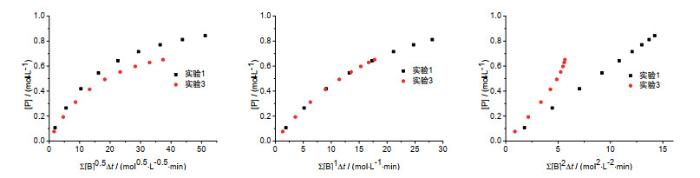## Introduction to a Novel Visual Method for the Treatment of Kinetic DataAbstract

Kinetic data treatment is one of the paramount contents of the kinetics in physical chemistry. There have been imperfections in the classical kinetic data treatment when faced with modern monitoring techniques which can supply extensive experimental data. Recently, variable time normalization analysis is raised to solve the above problems. With only several experiments under real experimental conditions, the rate constant and reaction order of the reactants and catalyst can be deduced by using visual comparison with the help of spreadsheet.

Keywords： Kinetic data ; Reaction order ; Integration method ; Visual method

Zhang Heng. Introduction to a Novel Visual Method for the Treatment of Kinetic Data. University Chemistry[J], 2021, 36(4): 2005032-0 doi:10.3866/PKU.DXHX202005032

## 1 方法简介

${\text{A}} + {\text{B}}\xrightarrow{{\text{C}}}{\text{P}} ( r{\text{ }} = {\text{ }}k{[\text{C}]^{0.5}}{[\text{A}]^1}{[\text{B}]^1} )$

 实验 [A]0/(mol∙L-1) [B]0/(mol∙L-1) [C]0/(mol∙L-1) 1 1.0 1.0 0.01 2 0.7 1.0 0.01 3 1.0 0.7 0.01 4 1.0 1.0 0.05

 t/min [A]/(mol∙L-1) [B]/(mol∙L-1) [P]/(mol∙L-1) Exp1 Exp2 Exp3 Exp4 Exp1 Exp2 Exp3 Exp4 Exp1 Exp2 Exp3 Exp4 0 1.000 0.7000 1.000 1.000 1.000 1.000 0.7000 1.000 0 0 0 0 2 0.89286 0.62378 0.92378 0.78844 0.89286 0.92378 0.62378 0.78844 0.10714 0.07622 0.07622 0.21156 6 0.73529 0.50719 0.80719 0.55402 0.73529 0.80719 0.50719 0.55402 0.26471 0.19281 0.19281 0.44598 12 0.5814 0.3881 0.6881 0.38315 0.5814 0.6881 0.3881 0.38315 0.4186 0.3119 0.3119 0.61685 20 0.45455 0.28637 0.58637 0.2715 0.45455 0.58637 0.28637 0.2715 0.54545 0.41363 0.41363 0.7285 30 0.35714 0.20669 0.50669 0.19901 0.35714 0.50669 0.20669 0.19901 0.64286 0.49331 0.49331 0.80099 42 0.28409 0.14688 0.44688 0.15072 0.28409 0.44688 0.14688 0.15072 0.71591 0.55312 0.55312 0.84928 56 0.22936 0.10294 0.40294 0.11746 0.22936 0.40294 0.10294 0.11746 0.77064 0.59706 0.59706 0.88254 72 0.18797 0.07107 0.37107 0.09381 0.18797 0.37107 0.07107 0.09381 0.81203 0.62893 0.62893 0.90619 90 0.15625 0.04824 0.34824 0.07648 0.15625 0.34824 0.04824 0.07648 0.84375 0.65176 0.65176 0.92352

## 2 方法原理及处理过程

$\begin{array}{l}\mathrm{d}[\mathrm{P}]=k[\mathrm{C}]^{\gamma}[\mathrm{B}]^{\beta}[\mathrm{A}]^{\alpha} \mathrm{d} t \end{array}$

$\mathrm{~d} f(t)=[\mathrm{A}]^{\alpha} \mathrm{d} t$

$\mathrm{~d}[\mathrm{P}]=k[\mathrm{C}]^{\gamma}[\mathrm{B}] \mathrm{d} t$

$f\left(t_{n}\right)=\int_{0}^{t_{n}}[\mathrm{~A}]^{\alpha} d t \approx \sum\limits_{i=1}^{n}\left(\frac{[\mathrm{A}]_{i}+[\mathrm{A}]_{i-1}}{2}\right)^{\alpha}\left(t_{i}-t_{i-1}\right)=\sum\limits_{i=1}^{n}[\mathrm{~A}]_{i}^{\alpha} \Delta t_{i}$

### 图1### 图2$\mathrm{d} g(t)=[\mathrm{A}]^{\alpha}[\mathrm{B}]^{\beta}[\mathrm{C}]^{\gamma} \mathrm{d} t$

### 图3$g({t_n}){\text{ = }}\int\limits_{\text{0}}^{{t_n}} {{{[\text{A}]}^\alpha }{{[\text{B}]}^\beta }{{[\text{C}]}^\gamma }\operatorname{d} t} \approx \sum\limits_{i = 1}^n {{{(\frac{{{{[\text{A]}]}_i} + {{[\text{A]}]}_{i - 1}}}}{2})}^\alpha }{{(\frac{{{{[{\text{B}}]}_i} + {{[{\text{B}}]}_{i - 1}}}}{2})}^\beta }{{[\text{C}]}^\gamma }({t_i} - {t_{i - 1}})} = \sum\limits_{i = 1}^n {[\text{A]}]_i^\alpha [\text{B}]_i^\beta {{[\text{C}]}^\gamma }\Delta {t_i}}$

$\mathrm{d}[\mathrm{P}]=k \mathrm{~d} g(t)$

### 图4## 参考文献 原文顺序 文献年度倒序 文中引用次数倒序 被引期刊影响因子

Burés J. Angew. Chem. Int. Ed. 2016, 55, 2028.

Burés J. Angew. Chem. Int. Ed. 2016, 55, 16084.

Martinez-Carrion A. ; Howlett M. G. ; Alamillo-Ferrer C. ; Clayton A. D. ; Bourne R. A. ; Codina A. ; Vidal-Ferran A. ; Adams R. W. ; Burés J. Angew. Chem. Int. Ed. 2019, 58, 10189.

Nielsen C. D. T. ; Burés J. Chem. Sci. 2019, 10, 348.

/

 〈〉